# Python小白的数学建模课-B6. 新冠疫情 SEIR 改进模型

SEIR 模型考虑存在易感者、暴露者、患病者和康复者四类人群，适用于具有潜伏期、治愈后获得终身免疫的传染病。

『Python小白的数学建模课 @ Youcans』 带你从数模小白成为国赛达人。

## 1. SEIR 基本模型

### 1.1 SEIR 模型的结构

SEIR 模型考虑存在易感者（Susceptible）、暴露者（Exposed）、患病者（Infectious）和康复者（Recovered）四类人群，适用于具有潜伏期、治愈后获得终身免疫的传染病。易感者（S 类）被感染后成为潜伏者（E类），随后发病成为患病者（I 类），治愈后成为康复者（R类）。这种情况更为复杂，也更为接近实际情况。

SEIR 模型的仓室结构示意图如下：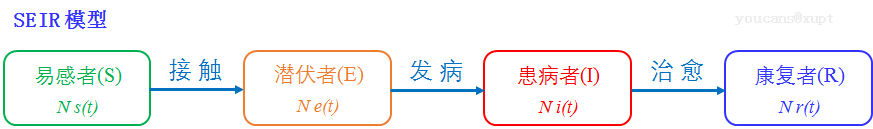### 1.2 SEIR 模型的假设

1. 考察地区的总人数 N 不变，即不考虑生死或迁移；

2. 人群分为易感者（S 类）、暴露者（E 类）、患病者（I 类）和康复者（R 类）四类；

3. 易感者（S 类）与患病者（I 类）有效接触即变为暴露者（E 类），暴露者（E 类）经过平均潜伏期后成为患病者（I 类）；患病者（I 类）可被治愈，治愈后变为康复者（R 类）；康复者（R类）获得终身免疫不再易感；

4. 将第 t 天时 S 类、E 类、I 类、R 类人群的占比记为 $s(t)$$e(t)$$i(t)$$r(t)$，数量分别为 $S(t)$$E(t)$$I(t)$$R(t)$；初始日期 $t=0$ 时，各类人群占比的初值为 $s_0$$e_0$$i_0$$r_0$

5. 日接触数 $\lambda$，每个患病者每天有效接触的易感者的平均人数；

6. 日发病率 $\delta$，每天发病成为患病者的暴露者占暴露者总数的比例；

7. 日治愈率 $\mu$，每天被治愈的患病者人数占患病者总数的比例，即平均治愈天数为 $1/\mu$

8. 传染期接触数 $\sigma = \lambda / \mu$，即每个患病者在整个传染期内有效接触的易感者人数。

### 1.3 SEIR 模型的微分方程

\begin{cases} \begin{align*} & \frac{ds}{dt} = -\lambda s i, &s(0)=s_0\\ & \frac{de}{dt} = \lambda s i - \delta e, &e(0)=e_0\\ & \frac{di}{dt} = \delta e - \mu i, &i(0)=i_0 \end{align*} \end{cases}

## 2. 基于 SEIR 模型研究新冠疫情

### 2.1 基于 SEIR 模型的新冠疫情研究论文

2019年12月，武汉市出现新冠疫情（COVID-19）病例；2020年初，新冠疫情（COVID-19）在中国迅速蔓延。随着严格的防疫措施，新冠疫情在中国总体被基本抑制；之后虽然在国内部分地区有零星散发，但均较快得到控制，这都得益于疫情早期的防控。

2020年1月，英国 Jonathan 等估计武汉市 2月4日感染病例将达到 19万例，高估了疫情发展态势。2020年1月，西安交通大学 Shen 等估计新冠疫情的基本再生数，预测最终感染人数在 2万人以内，明显低于公布的疫情数据。2020年1月31日，香港学者 Wu 等推测 1月25日感染人数超过6000，高于25日公布的确诊人数 1985例。

2020年 3月，钟南山院士团队在《Journal of thoracic disease》发表论文 “Modified SEIR and AI prediction of the epidemics trend of COVID-19 in China under public health interventions（ 基于改进 SEIR 和 AI 模型对公共卫生干预下的 COVID-19 暴发趋势预测）“，采用改进的 SEIR 模型来预测新冠疫情的发展。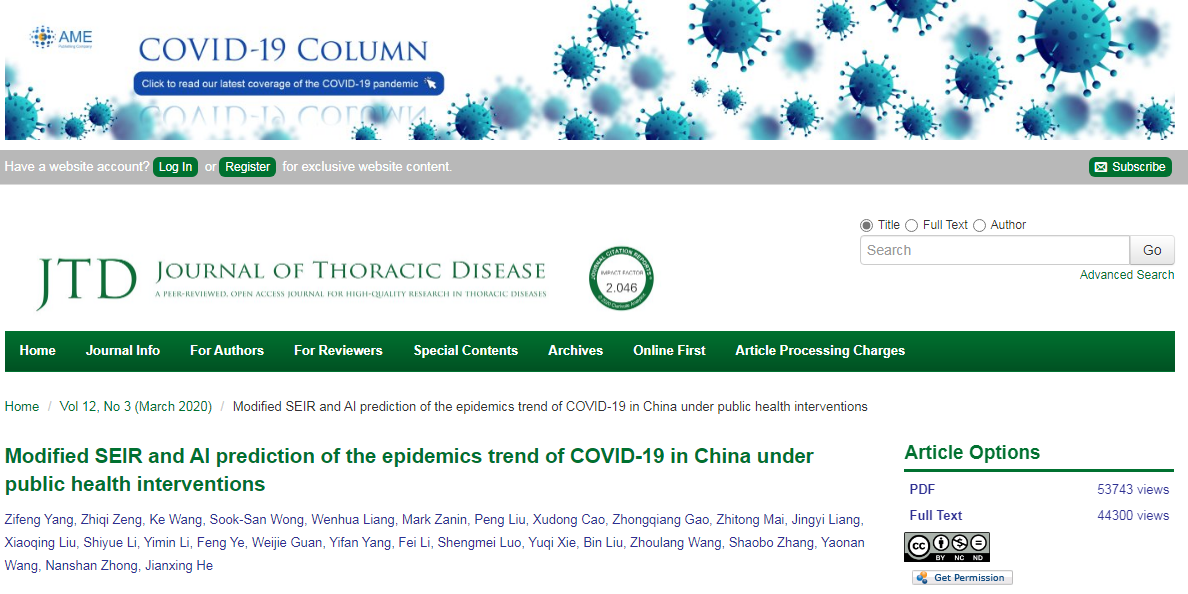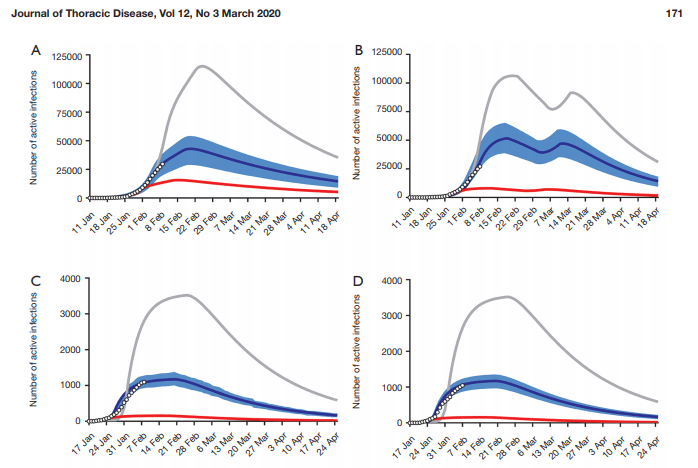2020年 2月，中山大学胡延庆团队在《科学通报》发表论文 “新型冠状病毒传播的数学模型与预测”，采用分阶段滚动 SEIR 模型对防控措施的效果进行分析，通过新冠疫情数据估算自然基本再生数为 2.57，对疫情发展趋势进行了预测，预计最终全国除湖北省以外的累计感染人数近 14000（到2020年底实际为 18922），除武汉市以外的累计感染人数近 32000（到2020年底实际为 36717）。

2020年 2月，西安交通大学吕军团队论文 “基于 SEIR模型分析相关干预措施在新型冠状病毒肺炎疫情中的作用”，对 SEIR模型加入潜伏期传染率、感染人群变化率等参数，通过新冠疫情数据估算基本再生数为 2.4～2.7。基于模型分析防控手段的有效性，模型显示基于严格限制出行的隔离措施能够减缓疫情发展的趋势，使潜伏和感染人群的峰值分别降低 45. 7%、29. 9%。因此，疫情一旦暴发应及时采取相应等级的应急响应措施，及时出台强力的管控举措，及早限制出行、居家隔离、提示及强制出行戴口罩等防护措施切断病毒传染途径，大幅度减少潜伏和感染人群与易感人群的接触人数，以减缓疫情发展及减少疫情峰值发病人数。

### 2.2 针对新冠疫情的 SEIR 模型改进

SEIR 模型的假设比 SI、SIS、SIR 模型更加复杂，也更符合实际情况，因而模型结果往往也与实际数据更为接近。但即便如此，相对于特定传染病、实际疫情的具体情况，SEIR 模型的基本假设仍然存在问题与不足。

SIR 模型较 SI 模型增加了康复者（R类），SEIR 模型较 SIR模型增加了潜伏者（E类）。类似地，结合新冠疫情传播和防控的特征，可以而且需要对人群进行进一步的细分。

“早发现、早诊断、早隔离、早治疗”是新冠疫情防控的关键措施。尽早发现患病者和尚未发病的潜伏者，对其进行隔离，可以大幅降低日接触数、传染期接触数。因此，潜伏者处于检出后的隔离状态还是未检出的正常活动状态，对于疫情传播的影响是具有本质差异的，由此可以在 SEIR模型中引入“隐形传播者（Undiscovered）”。

SEIR 模型是单向模型，是对实际问题的简化，便于分析和求解。考虑新冠疫情发病机制和传播特点，可以对各类人群之间的传播特性进行更科学、更细致的研究。

## 3. 考虑潜伏期传染性的 SEIR 改进模型

### 3.1 改进模型的假设和微分方程

1. 潜伏者日接触数 $\lambda_2$，每个潜伏者每天有效接触的易感者的平均人数。

\begin{align} & N \frac{ds}{dt} = - N \lambda s i - N \lambda_2 s e\\ & N \frac{de}{dt} = N \lambda s i + N \lambda_2 s e - N \delta e\\ & N \frac{di}{dt} = N \delta e - N \mu i\\ & N \frac{dr}{dt} = N \mu i\\ \end{align}

\begin{cases} \begin{align*} & \frac{ds}{dt} = -\lambda s i - \lambda_2 s e, &s(0)=s_0\\ & \frac{de}{dt} = \lambda s i + \lambda_2 s e - \delta e, &e(0)=e_0\\ & \frac{di}{dt} = \delta e - \mu i, &i(0)=i_0 \end{align*} \end{cases}

### 3.2 odeint() 求解 SEIR 模型的编程步骤

1. 导入 scipy、numpy、matplotlib 包。
2. 定义导数函数 $f(y,t)$。改进模型只是在 SEIR 模型微分方程中增加了一个修正项，具体编程并没有很大区别，以下给出例程以供对比。

SEIR 模型的微分方程导数函数

def dySEIR(y, t, lamda, delta, mu):  # SEIR 模型，导数函数
s, e, i = y
ds_dt = - lamda*s*i  # ds/dt = -lamda*s*i
de_dt = lamda*s*i - delta*e  # de/dt = lamda*s*i - delta*e
di_dt = delta*e - mu*i  # di/dt = delta*e - mu*i
return np.array([ds_dt,de_dt,di_dt])


SEIR 改进模型的微分方程导数函数

def dySEIR2(y, t, lamda, lam2, delta, mu):  # SEIR2 模型，导数函数
s, e, i = y
ds_dt = - lamda*s*i - lam2*s*e # ds/dt = -lamda*s*i - lam2*s*e
de_dt = lamda*s*i + lam2*s*e - delta*e  # de/dt = lamda*s*i - delta*e
di_dt = delta*e - mu*i  # di/dt = delta*e - mu*i
return np.array([ds_dt,de_dt,di_dt])


Python 可以直接对向量、向量函数进行定义和赋值，使程序更为简洁。但考虑读者主要是 Python 小白，又涉及到看着就心烦的微分方程组，所以我们宁愿把程序写得累赘一些，便于读者将程序与前面的微分方程组逐项对应。

1. 定义初值 $y_0$$y$ 的定义区间 $[t_0,\ t]$，注意初值为数组向量 $y_0=[s_0,e_0,i_0]$
2. 调用 odeint() 求 $y$ 在定义区间 $[t_0,\ t]$ 的数值解。

### 3.3 Python 例程：考虑潜伏期传染性的 SEIR 改进模型

# modelCovid5_v1.py
# Demo01 of mathematical modeling for Covid2019
# Improved SEIR model for epidemic diseases (改进的 SEIR 模型)
# Crated：2021-06-16
# Python小白的数学建模课 @ Youcans

# 1. SEIR2 模型，考虑潜伏期具有传染性
from scipy.integrate import odeint  # 导入 scipy.integrate 模块
import numpy as np  # 导入 numpy包
import matplotlib.pyplot as plt  # 导入 matplotlib包

def dySEIR(y, t, lamda, delta, mu):  # SEIR 模型，导数函数
s, e, i = y
ds_dt = - lamda*s*i  # ds/dt = -lamda*s*i
de_dt = lamda*s*i - delta*e  # de/dt = lamda*s*i - delta*e
di_dt = delta*e - mu*i  # di/dt = delta*e - mu*i
return np.array([ds_dt,de_dt,di_dt])

def dySEIR2(y, t, lamda, lam2, delta, mu):  # SEIR2 模型，导数函数
s, e, i = y
ds_dt = - lamda*s*i - lam2*s*e # ds/dt = -lamda*s*i - lam2*s*e
de_dt = lamda*s*i + lam2*s*e - delta*e  # de/dt = lamda*s*i - delta*e
di_dt = delta*e - mu*i  # di/dt = delta*e - mu*i
return np.array([ds_dt,de_dt,di_dt])

# 设置模型参数
number = 1e5  # 总人数
lamda = 1.0  # 日接触率, 患病者每天有效接触的易感者的平均人数
lam2 = 0.25  # 日接触率2, 潜伏者每天有效接触的易感者的平均人数
delta = 0.05  # 日发病率，每天发病成为患病者的潜伏者占潜伏者总数的比例
mu = 0.05  # 日治愈率, 每天治愈的患病者人数占患病者总数的比例
sigma = lamda / mu  # 传染期接触数
fsig = 1-1/sigma
tEnd = 200  # 预测日期长度
t = np.arange(0.0, tEnd, 1)  # (start,stop,step)
i0 = 1e-3  # 患病者比例的初值
e0 = 0  # 潜伏者比例的初值
s0 = 1-i0  # 易感者比例的初值
Y0 = (s0, e0, i0)  # 微分方程组的初值

# odeint 数值解，求解微分方程初值问题
ySEIR = odeint(dySEIR, Y0, t, args=(lamda,delta,mu))  # SEIR 模型
ySEIR2 = odeint(dySEIR2, Y0, t, args=(lamda,lam2,delta,mu))  # SEIR2 模型

# 输出绘图
print("lamda={}\tmu={}\tsigma={}\t(1-1/sig)={}".format(lamda,mu,sigma,fsig))
plt.title("Comparison between SEIR and improved SEIR model")
plt.xlabel('t-youcans')
plt.axis([0, tEnd, -0.1, 1.1])

plt.plot(t, ySEIR2[:,0], '-g', label='s(t)-iSEIR')  # 易感者比例
plt.plot(t, ySEIR2[:,1], '-b', label='e(t)-iSEIR')  # 潜伏者比例
plt.plot(t, ySEIR2[:,2], '-m', label='i(t)-iSEIR')  # 患病者比例
# plt.plot(t, 1-ySEIR2[:,0]-ySEIR2[:,1]-ySEIR2[:,2], '-b', label='r(t)-iSEIR')
plt.plot(t, ySEIR[:,0], '--g', label='s(t)-SEIR')
plt.plot(t, ySEIR[:,1], '--b', label='e(t)-SEIR')
plt.plot(t, ySEIR[:,2], '--m', label='i(t)-SEIR')
# plt.plot(t, 1-ySEIR[:,0]-ySEIR[:,1]-ySEIR[:,2], '--m', label='r(t)-SEIR')
plt.legend(loc='upper right')  # youcans
plt.show()



### 3.4 SEIR 改进模型的结果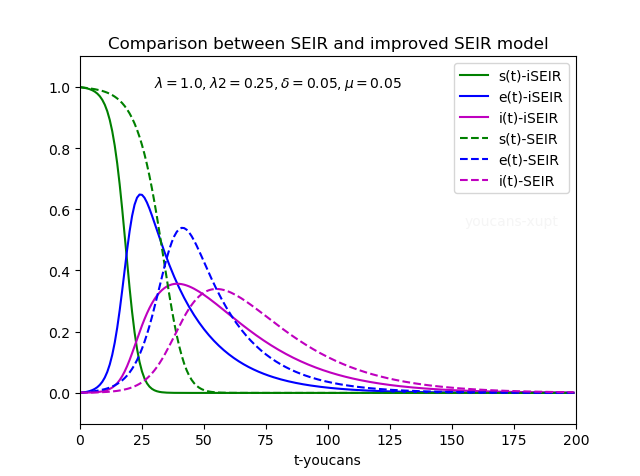## 4. 基于 SEIR 改进模型的防控措施分析

### 4.1 对患病者实施隔离措施的影响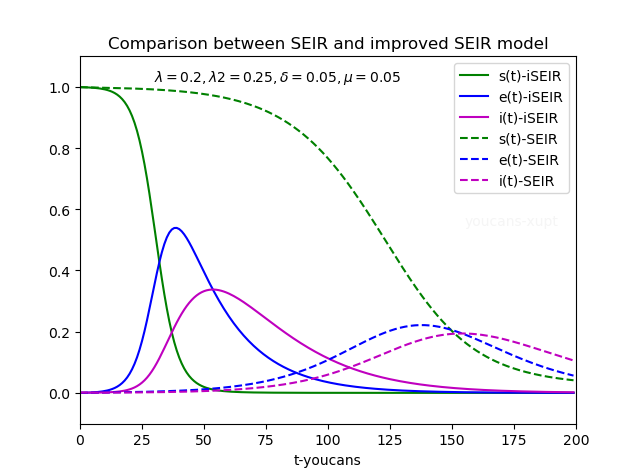### 4.2 对潜伏者实施隔离措施的影响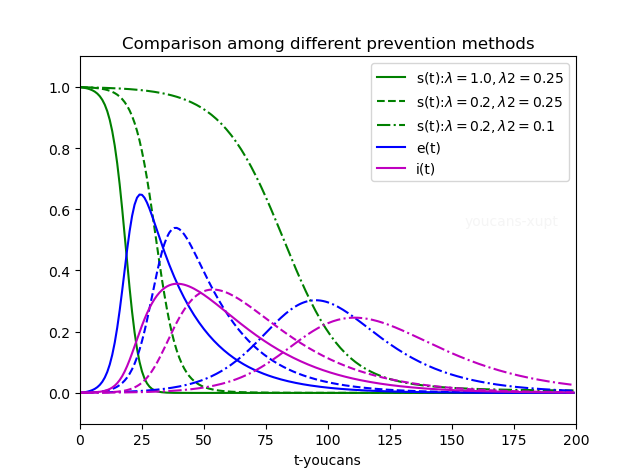## 5. 总结

• 改进模型通常是针对特定传染病和实际疫情的具体情况，在基本模型的基础上考虑新的因素，使模型的假设更符合实际情况，模型的结果能接近实际数据，才能更准确地预测疫情发展趋势、分析防控措施的影响。
• 结合新冠疫情对 SEIR 模型改进的主要方向是：对人群类型的细分；对疫情传播特征的修正；对模型基本假设的完善。
• 以考虑潜伏期传染性的 SEIR 改进模型为例，给出了具体的数学模型、编程实现、结果讨论。
• 从新冠疫情建模的角度分析看，需要考虑潜伏期传染性对疫情传播的影响；从新冠疫情防控的角度看，对潜伏者早发现、早诊断、早隔离，对于疫情防控是有效和必要的。

【本节完】

1. 钟南山 等，Modified SEIR and AI prediction of the epidemics trend of COVID-19 in China under public health interventions（ 基于改进 SEIR 和 AI 模型对公共卫生干预下的 COVID-19 暴发趋势预测），Journal of the thoracic disease，2020.3
2. 谢家荣 等，新型冠状病毒传播的数学模型与预测，科学通报，2020，65(22)
3. 林俊锋，基于引入隐形传播者的 SEIR 模型的 COVID-19 疫情分析和预测，电子科技大学学报，2020，49(3)
4. 曹盛力 等，修正 SEIR 传染病动力学模型应用于湖北省2019冠状病毒病（COVID-19）疫情预测和评估，浙江大学学报（医学版），2020.4
5. 耿辉 等，基于 SEIR模型分析相关干预措施在新型冠状病毒肺炎疫情中的作用，暨南大学学报，2020,41(2)
6. 王思远 等，基于改进传染病动力学易感-暴露-感染-恢复模型 (SEIR) 预测新型冠状病毒肺炎疫情，第二军医大学学报，2020，41(6)
7. 王晨曦 等，传染病动力学模型研究综述，中国计算机用户协会网络应用分会2020年第二十四届网络新技术与应用年会论文集，2020.12
8. 董是 等，基于系统动力学模型的 2019冠状病毒病早期防控机制研究，浙江大学学报（医学版），2021.2